# 使用pandas筛选出指定列值所对应的行

2019/04/10 10:10

``````select * from table where column_name = some_value;
``````

pandas中获取数据的有以下几种方法：

• 布尔索引
• 位置索引
• 标签索引
• 使用API

``````import pandas as pd
import numpy as np

df = pd.DataFrame({'A': 'foo bar foo bar foo bar foo foo'.split(),
'B': 'one one two three two two one three'.split(),
'C': np.arange(8), 'D': np.arange(8) * 2})
``````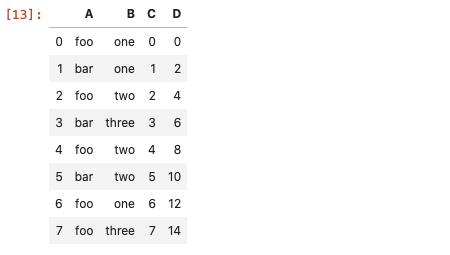##### 布尔索引

``````df[df['A'] == 'foo'] # 判断等式是否成立
``````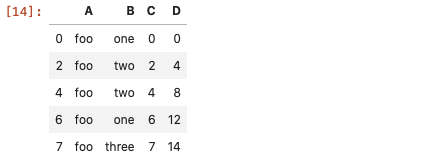##### 位置索引

``````mask = df['A'] == 'foo'
pos = np.flatnonzero(mask)  # 返回的是array([0, 2, 4, 6, 7])
df.iloc[pos]

#常见的iloc用法
df.iloc[:3,1:3]
``````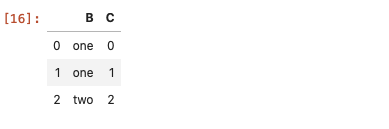##### 标签索引

``````df.set_index('A', append=True, drop=False).xs('foo', level=1) # xs方法适用于多重索引DataFrame的数据筛选

# 更直观点的做法
df.index=df['A'] # 将A列作为DataFrame的行索引
df.loc['foo', :]

# 使用布尔
df.loc[df['A']=='foo']
``````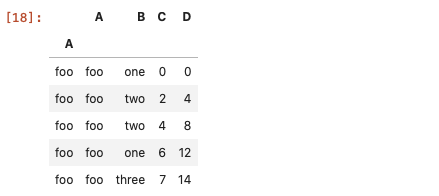##### 使用API

`pd.DataFrame.query`方法在数据量大的时候，效率比常规的方法更高效。

``````df.query('A=="foo"')

# 多条件
df.query('A=="foo" | A=="bar"')
``````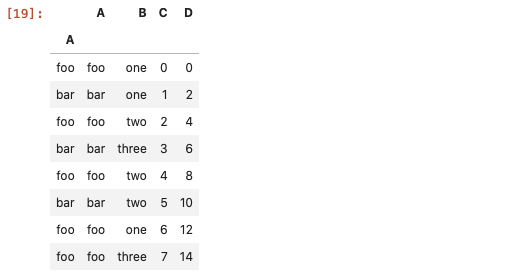``````df.loc[df['column_name'] == some_value]
``````

2、筛选出列值属于某个范围内的行，用isin

``````df.loc[df['column_name'].isin(some_values)]  # some_values是可迭代对象
``````

3、多种条件限制时使用&，&的优先级高于>=或<=，所以要注意括号的使用

``````df.loc[(df['column_name'] >= A) & (df['column_name'] <= B)]
``````

4、筛选出列值不等于某个/些值的行

``````df.loc[df['column_name'] != 'some_value']

df.loc[~df['column_name'].isin('some_values')] #~取反
``````0
0 收藏

### 作者的其它热门文章0 评论
0 收藏
0